### Information Measures

PROC ENTROPY returns several measures of fit. First, the value of the objective function is returned. Next, the signal entropy is provided followed by the noise entropy. The sum of the noise and signal entropies should equal the value of the objective function. The next two metrics that follow are the normed entropies of both the signal and the noise.

Normalized entropy (NE) measures the relative informational content of both the signal and noise components through p and w, respectively (Golan, Judge, and Miller, 1996). Let S denote the normalized entropy of the signal,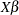, defined as: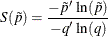where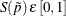. In the case of GME, where uniform priors are assumed, S can be written as: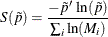where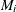is the number of support points for parameter. A value of 0 for S implies that there is no uncertainty regarding the parameters; hence, it is a degenerate situation. However, a value of 1 implies that the posterior distributions equal the priors, which indicates total uncertainty if the priors are uniform.

Because NE is relative, it can be used for comparing various situations. Consider adding a data point to the model. If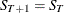, then there is no additional information contained within that data constraint. However, if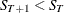, then the data point gives a more informed set of parameter estimates.

NE can be used for determining the importance of particular variables with regard to the reduction of the uncertainty they bring to the model. Each of theparameters that is estimated has an associated NE defined as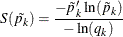or, in the GME case,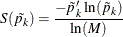where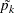is the vector of supports for parameter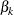and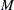is the corresponding number of support points. Since a value of 1 implies no relative information for that particular sample, Golan, Judge, and Miller (1996) suggest an exclusion criteria of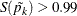as an acceptable means of selecting noninformative variables. See Golan, Judge, and Miller (1996) for some simulation results.

The final set of measures of fit are the parameter information index and error information index. These measures can be best summarized as 1 – the appropriate normed entropy.